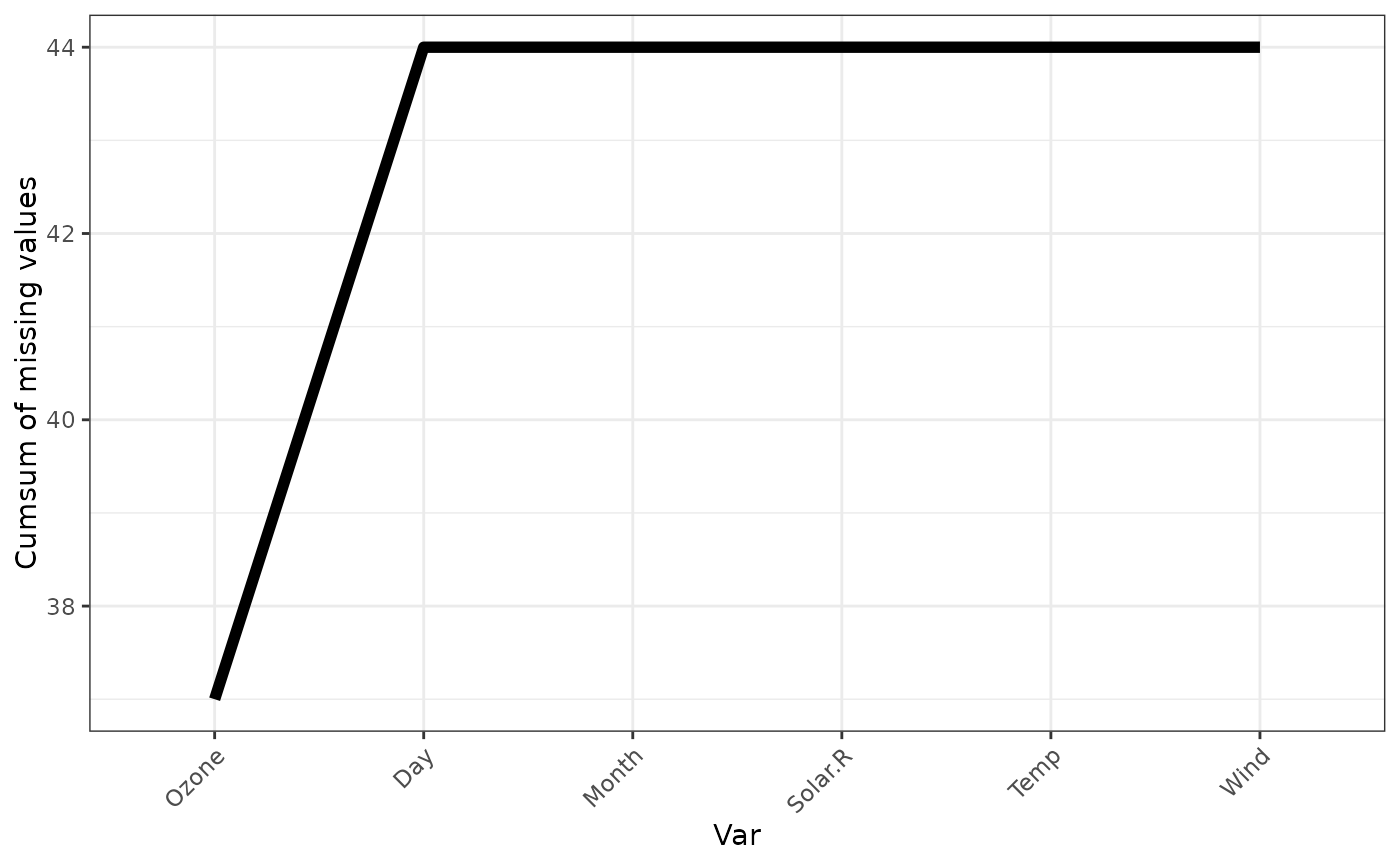A plot showing the cumulative sum of missing values for each variable, reading columns from the left to the right of the initial dataframe. A default minimal theme is used, which can be customised as normal for ggplot.

## Usage

gg_miss_var_cumsum(x)

x

a data.frame

## Value

a ggplot object showing the cumulative sum of missings over the variables

geom_miss_point() gg_miss_case() gg_miss_case_cumsum gg_miss_fct() gg_miss_span() gg_miss_var() gg_miss_which()